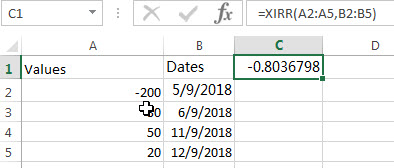# Excel XIRR Function

This post will guide you how to use Excel XIRR function with syntax and examples in Microsoft excel.

### Description

The Excel XIRR function returns the internal rate of return for a series of cash flows that is not necessarily periodic. And if you want to get the internal rate of return for a series of periodic cash flows, you can use the IRR function in Excel.

The XIRR function is a build-in function in Microsoft Excel and it is categorized as a Financial Function.

The XIRR function is available in Excel 2016, Excel 2013, Excel 2010, Excel 2007, Excel 2011 for Mac.

### Syntax

The syntax of the XIRR function is as below:

`= XIRR (values, dates, [guess])`

Where the XIRR function arguments are:

• Values -This is a required argument. An array or cell reference that contain numbers for which you want to calculate the internal rate of return. And the values must include at least one positive value and one negative value.
• Dates -This is a required argument. A series of dates that corresponds to the cash flow payments.
• Guess – This is an optional argument. An initial guess at the internal rate of return. If it is omitted, and it will be set as 0.1 or 10%.

Note:

• The values arguments must contain at least one positive value and one negative cash flow value, or the XIRR function will return the #NUM! Error.
• If you provide an invalid date in the date argument, the XIRR function will return the #NUM! Error.

### Excel XIRR Function Examples

The below examples will show you how to use Excel XIRR Function to calculate the internal rate of return for a series of cash flows.

#1 to get the internal rate of return, using the following formula:

`=XIRR(A2:A5,B2:B5)`### Related Functions

• Excel IRR Function
The Excel IRR function returns the internal rate of return for a series of cash flows and the cash flows must be occurred at regular intervals (monthly or annually).The syntax of the IRR function is as below:=IRR(values, [guess])…

### Related Examples

• Calculate Stock Rate of Return
You can try to calculate the rate of return by manually, or you use an Excel formula to achieve the result. The best way to calculate your rate of return is to use the EXCEL XIRR function,…
Related Posts

Calculate Stock Rate of Return

This post will discuss that how to calculate the rate of return for the share of stock in Excel. And to calculate the return for a share of stock, you need to know the starting price, ending price and dividends ...

Excel DSUM Function

This post will guide you how to use Excel DSUM function with syntax and examples in Microsoft excel. Description The Excel DSUM Function will add the numbers in a column or database that meets a given criteria. And so you ...

Excel DSTDEVP Function

This post will guide you how to use Excel DSTDEVP function with syntax and examples in Microsoft excel. Description The Excel DSTDEVP returns the standard deviation of a population based on the entire population of numbers in a column or ...

Excel DSTDEV Function

This post will guide you how to use Excel DSTDEV function with syntax and examples in Microsoft excel. Description The Excel DSTDEV returns the standard deviation of a population based on a sample of numbers in a column or database ...

Excel DMIN Function

This post will guide you how to use Excel DMIN function with syntax and examples in Microsoft excel. Description The Excel DMIN returns the minimum value from a database or a column of a list that matches the specified conditions. ...

Excel DPRODUCT Function

This post will guide you how to use Excel DPRODUCT function with syntax and examples in Microsoft excel. Description The Excel DPRODUCT returns the product of values from a set of records that match criteria that you specify. The DPRODUCT ...

Excel VDB Function

This post will guide you how to use Excel VDB function with syntax and examples in Microsoft excel. Description The Excel VDB function calculates the depreciation of an asset for a specified period based on the double declining balance method. ...

Excel SYD Function

This post will guide you how to use Excel SYD function with syntax and examples in Microsoft excel. Description The Excel SYD function calculates the sum-of-years’digits depreciation of an asset for a specified period. And it will return a numeric ...

Excel SLN Function

This post will guide you how to use Excel SLN function with syntax and examples in Microsoft excel. Description The Excel SLN function calculates the depreciation of an asset for one period based on the straight line depreciation. And it ...

Excel PV Function

This post will guide you how to use Excel PV function with syntax and examples in Microsoft excel. Description The Excel PV function returns the present value of a loan or investment based on constant payments and a constant interest ...

Sidebar